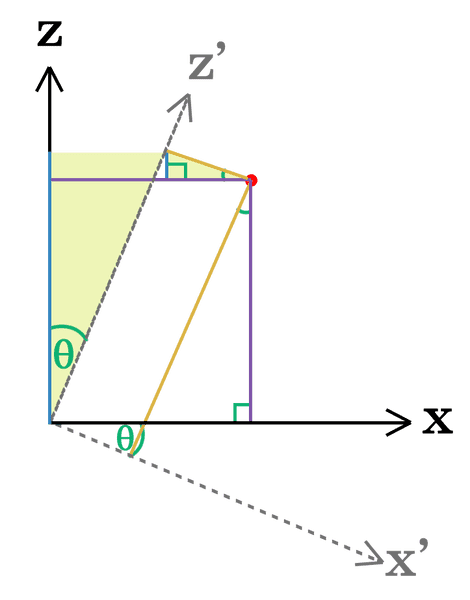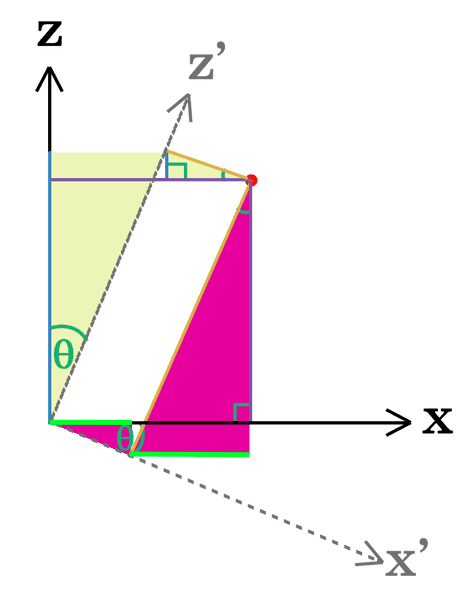# Rotation of a point in R3 about the y-axis

• I
Hello,

I'm having a visualisation problem. I have a point in R3 that I want to rotate about the ##y##-axis anticlockwise (assuming a right-handed cartesian coordinate system.) I know that the change to the point's ##x## and ##z## coordinates can be described as follows:

$$z = z'\cos\theta-x'\sin\theta$$
$$x = x'\cos\theta+z'\sin\theta$$

My problem is that I only seem to be able to know this "as fact" and I am trying to draw a visual aid to help me see how this has come about. I produced the following diagram: (I know the rotation looks clockwise but it's anticlockwise as the ##y##-axis is coming out of the page/screen)As you can see, the first equation for the ##z## coordinate of the point is derivable by looking at the heights (blue edges) of the pale yellow triangles. The longer blue edge is ##z'\cos\theta## by simple trig and the shorter one is ##x'\sin\theta##. Take the difference of the 2 to work out the ##z## coordinate (where the purple line touches the ##z##-axis) et voila.

The issue is I am struggling to come up with a similar visualisation for ##x##. I just can't find the right triangles to make that sum. I've burned through ~10 sheets of paper already. It's definitely one of those "need-to-disengage-to-see-clearly-again" situations because I have definitely done this before, but I figured someone on here might speed this tedious process up and guide me on how to visually represent the equation for ##x##. Either verbally point me in the right or scribble on top of my drawing, I'm not bothered. Thanks!

#### Attachments

BvU
Homework Helper
What makes you say the y axis is coming out of the screen ? Do you know about right-handed and left-handed coordinate systems ?

Filip Larsen
Gold Member
Another approach to understanding this could be to understand a more general exposition like Rodrigues' rotation formula  and then just apply the values that apply for your case. At least, for practical applications this a very handy equation.

 https://en.wikipedia.org/wiki/Rodrigues'_rotation_formula

mathman
Visual aid: ignore y. Set up graph with x and z as axes. Then put in a pair of perpendicular lines through the origin and label them x' and z'. Label the angle between x and x' as ##\theta## and compute the relationships

What makes you say the y axis is coming out of the screen ? Do you know about right-handed and left-handed coordinate systems ?
I was confused by your comment at first but you're right, I have it the wrong way around. OK, so the ##y##-axis (as per right-handed cartesian system) is going into the screen. The only way to make my diagram still relevant is to instead say that we're rotating clockwise around ##y##, so my little comment about that is completely irrelevant. Will edit the OP as necessary. EDIT: Seems I can't actually edit it any more.

Visual aid: ignore y. Set up graph with x and z as axes. Then put in a pair of perpendicular lines through the origin and label them x' and z'. Label the angle between x and x' as ##\theta## and compute the relationships
If you open the spoiler you will see that I had an attempt at exactly what you describe. Managed to get one (but not both) of the equations that way. This thread was made because I got stuck

Hi all, excuse the double post but since I just managed to find what I was looking for I thought I'd share in case anybody else finds seeing where the equations in the OP come from

Quick scribble since I can't be bothered to make it as neat as my original diagram at the moment:The ##x##-coordinate is obtained by adding the 2 widths (neon green lines) of the fuchsia triangles.

#### Attachments

PeroK
Homework Helper
Gold Member
2020 Award
Hi all, excuse the double post but since I just managed to find what I was looking for I thought I'd share in case anybody else finds seeing where the equations in the OP come from

A couple of observations. You have taken a point in the ##x'-z'## system and calculated its coordinates in the ##x-z## system. Effectively, you have rotated your axes by ##\theta##. The primed axes are the unprimed axes rotated clockwise by ##\theta##.

This gives the same form of the equations as taking a vector in the ##x-z## system, with coordinates ##(x, z)##, and rotating it anticlockwise by ##\theta## and giving the coordinates of the new vector as ##(x', z')##.

Note that the simplest way to generate the coordinates is to use the sum of angles formulae for sine and cosine. If a vector has a polar angle of ##\alpha## anticlockwise from the x-axis and is rotated by an angle ##\theta## anticlockwise, then its new polar angle is ##\alpha + \theta##. If you expand the expressions for ##\cos(\alpha + \theta)## and ##\sin(\alpha + \theta)##, you get the required formula for the coordinates of a rotated vector.